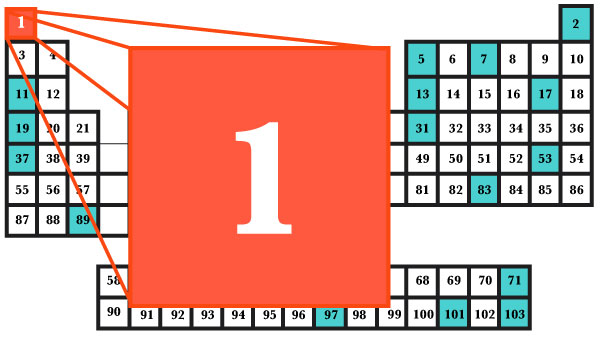# Integer chemistry II

Level 2Integers, like molecules, are built from atoms. However there's only one element in the periodic table of maths, namely the number 1, and all other integers can be built from it. For example, there are several ways to build the number 6:

$6 = 1+1+1+1+1+1 \\ = (1+1)*(1+1)+1+1 \\ = (1+1)*(1+1+1)$

We say the number 6 has complexity 5, because that's the cheapest (using the fewest ones) way it can be built. The allowed operations are addition, multiplication and brackets. It's not allowed to write two ones next to each other to make 11.

What's the complexity of the number 31?

×

Problem Loading...

Note Loading...

Set Loading...